# Polyform Database

Data for some of the most popular polyforms

## Examples

### Basic Examples

Retrieve the resource:

 In:=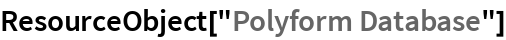Out=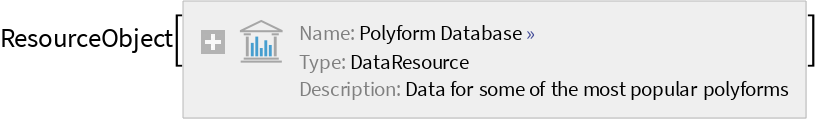Retrieve a sample of the default content:

 In:=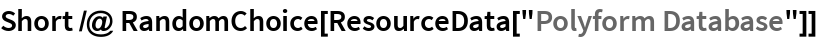Out=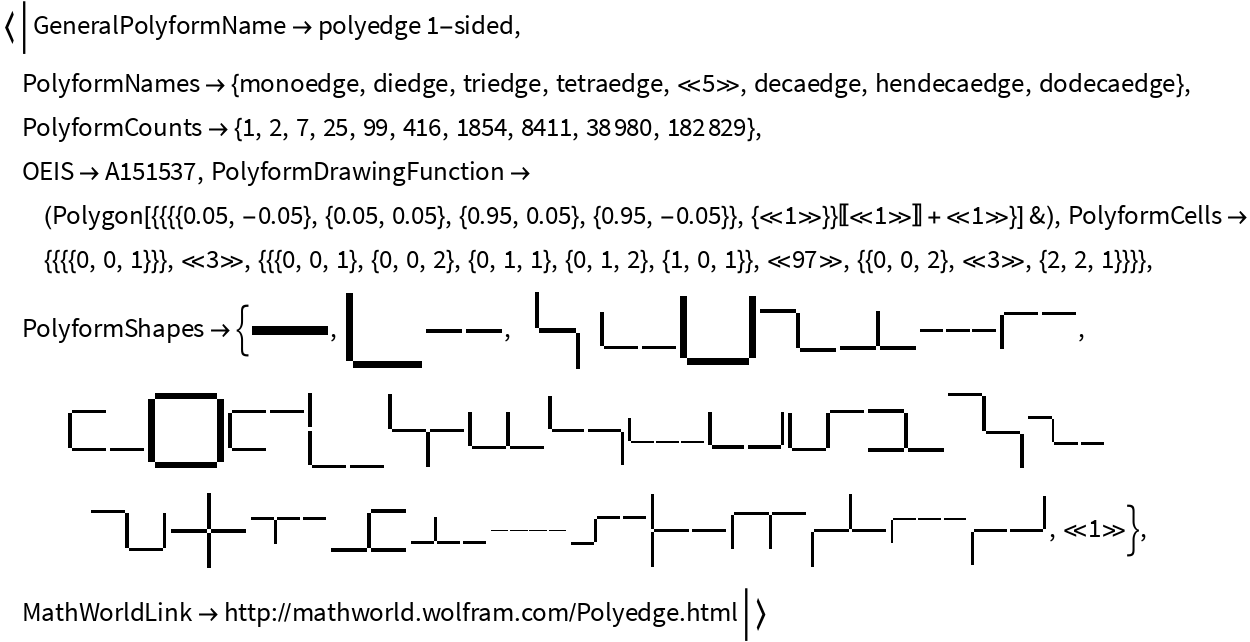Show the shapes for a polyform:

 In:=Out=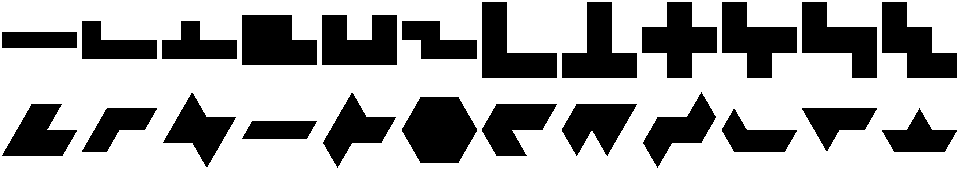The number of polyforms of a given type and order is given in PolyformCounts:

 In:=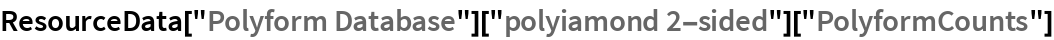Out=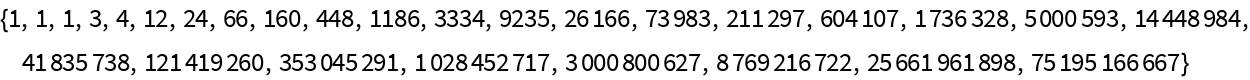A coordinate form for all polyforms is given. Component cells can have different orientations, so a drawing function is included for each polyform:

 In:=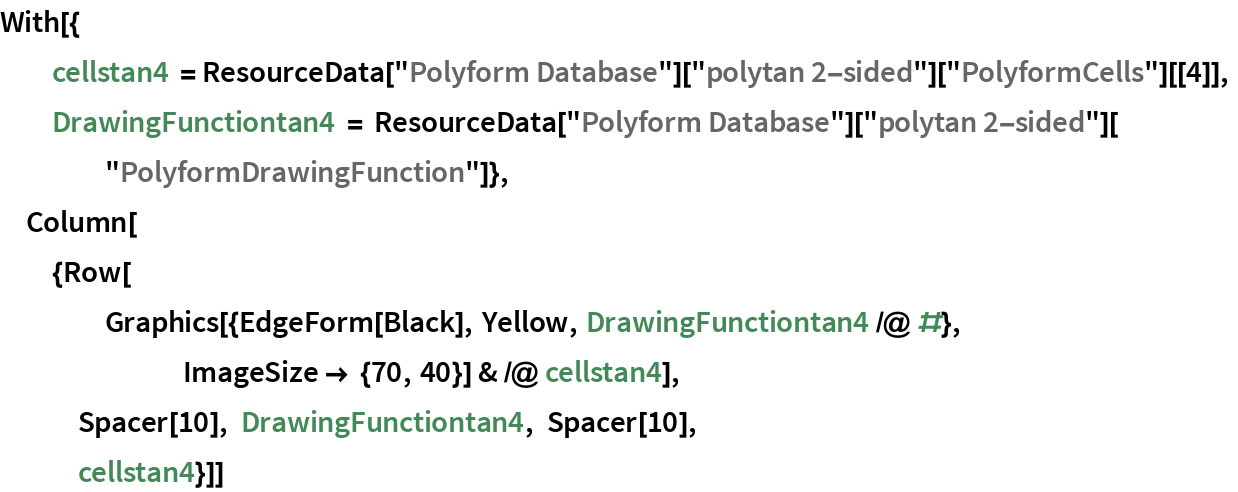Out=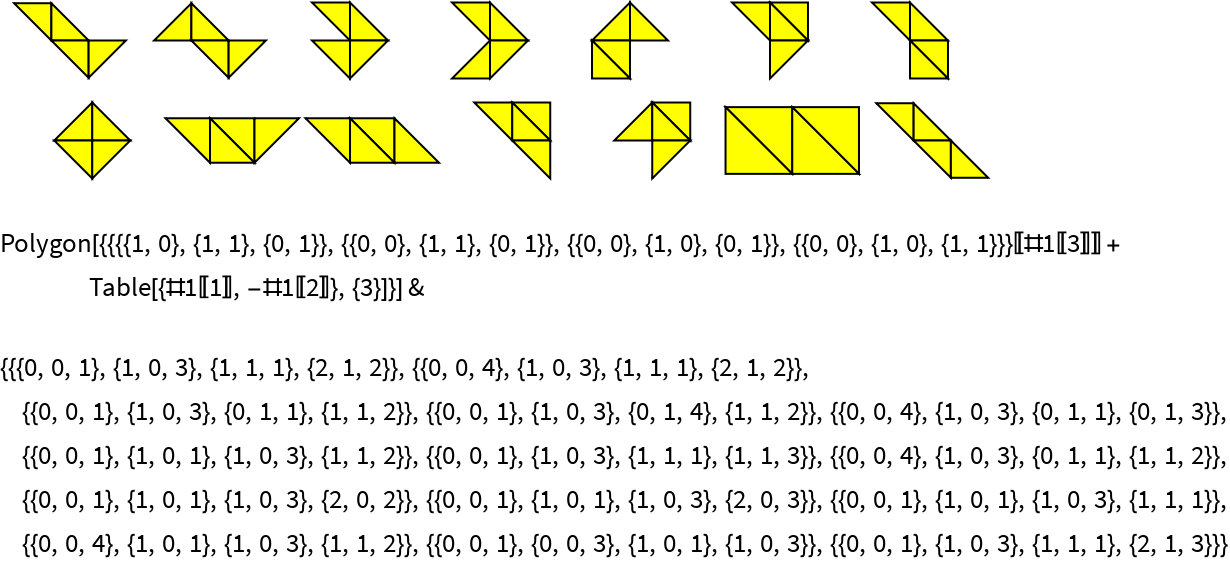In:=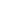Wolfram Research, "Polyform Database" from the Wolfram Data Repository (2017) https://doi.org/10.24097/wolfram.40377.data